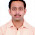## Monday, October 16, 2006

### Favorite Theorems: The Yao Principle

September Edition

What is the best a probabilistic algorithm can do for the worst-case input? Perhaps it might be easier to show the limitations of a deterministic algorithm on the average over an adversarially chosen distribution of inputs. Andrew Yao observed these values are one and the same.

Andrew Yao, "Probabilistic Computations: Toward a Unified Measure of Complexity." FOCS 1977

Best to view this result as a zero-sum game. Alice chooses a deterministic algorithm A and Ian chooses an input I. Ian receives t dollars from Alice where t is the "cost" of algorithm A on input I. By applying von Neumann's minimax theorem Ian and Alice have probabilistic equilibrium strategies. That is Ian has a distribution of inputs that achieve an expected cost t no matter what algorithm Alice chooses. Also Alice has a probabilistic algorithm (a randomized choice of deterministic algorithms) that will achieve an expected cost of the same t no matter what input Ian chose.

The Yao Principle applies when we don't consider the algorithmic complexity of the players. For example in communication complexity we have two players who each have a separate half of an input string and they want to compute some function of the input with the minimum amount of communication between them. The Yao principle states that the best probabilistic strategies for the players will achieve exactly the communication bounds as the best deterministic strategy over a worst-case distribution of inputs.

The Yao Principle plays a smaller role where we measure the running time of an algorithm since applying the Principle would require solving an extremely large linear program. But since so many of our bounds are in information-based models like communication and decision-tree complexity, the Yao Principle, though not particularly complicated, plays an important role in lower bounds in a large number of results in our field.

1.One of my favorite as well. I would add that this is very easy to use: instead of finding a single worst case (say, for a fixed size of input), you just have to find a bunch of those "worst cases", forming a distribution large enough that no algorithm can take advantage of it. Typically, taking all the instances which are the worst case of some deterministic algorithm...

2.This is regularly referred to as Yao's principle, and hailed as a great result. What I never understood was, is this -really- more than an application of Von Neumann's minimax theorem? It seems like a corollary od Von Neumman's theorem, that gets huge amounts of credit.

3.To anonymous:

As Lance said "Andrew Yao observed..."

Even though the result is trivial to prove, it is amazingly important and useful.

I believe this is why Yao gets so much credit for it.

(I am just a student. Some famous complexity theorist should back me up..)

4.Famous C. Theorist11:12 AM, October 17, 2006

I am a famous complexity theorist and I approve the previous message.

5.To Anonymous,
its not that how easy to prove matters. It is how he modelled and observed. Like, Nash theorem is just application of kakatuni's fix point theorem. Credit goes for the modelling so that it is easy to prove and really works.

6.To I am what I am/Anonymous:

In fact, when Nash went to von Neumann to present his theorem, he was dismissed with 'But that's just a fixed point theorem, it's trivial!'. That, however, won a Nobel.